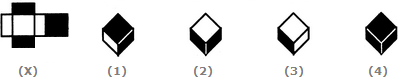# Non Verbal Reasoning - Cubes and Dice - Discussion

### Discussion :: Cubes and Dice - Section 1 (Q.No.18)

The sheet of paper shown in the figure (X) given on the left hand side, in each problem, is folded to form a box. Choose from amongst the alternatives (1), (2), (3) and (4), the boxes that are similar to the box that will be formed.

18.

Choose the box that is similar to the box formed from the given sheet of paper (X).[A]. 1 and 3 only [B]. 2 and 4 only [C]. 3 and 4 only [D]. 1 and 4 only

Explanation:

The fig. (X) is similar to Form I. So, when the sheet shown in fig. (X) is folded to form a box (cuboid), then the two rectangular-shaded faces lie opposite to each other, two rectangular white faces lie opposite to each other and the two square shaped faces (one shaded and one white) lie opposite to each other. Clearly, the cuboids shown in figures (2) and (4) cannot be formed as in each of the two cuboids the two shaded rectangular faces appear adjacent to each other. So, only the cuboids in figures (1) and (3) can be formed.